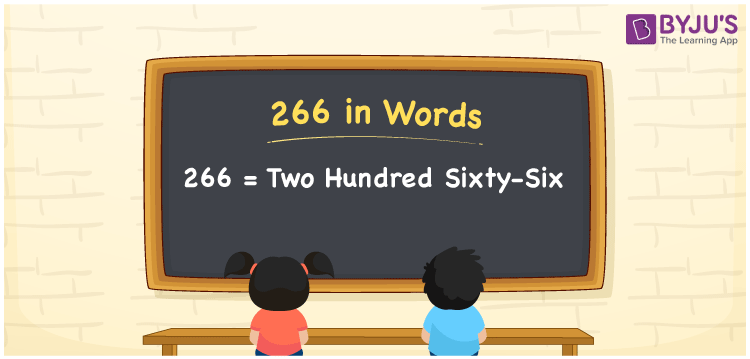# 266 in words

266 in words is written as Two Hundred and Sixty Six. 266 represents the count or value. The article on Counting Numbers can give you an idea about count or counting. The number 266 is used in expressions that relate to money, days, distance, length, weight and so on. Let us consider an example for 266. “The bus number Two Hundred and Sixty Six should arrive at any time now.”

 266 in words Two Hundred and Sixty Six Two Hundred and Sixty Six in Numbers 266

## 266 in English Words## How to Write 266 in Words?

We can convert 266 to words using a place value chart. This can be done as follows. The number 266 has 3 digits, so let’s make a chart that shows the place value up to 3 digits.

 Hundreds Tens Ones 2 6 6

Thus, we can write the expanded form as:

2 × Hundred + 6 × Ten + 6 × One

= 2 × 100 + 6 × 10 + 6 × 1

= 266

= Two Hundred and Sixty Six.

266 is the natural number that is succeeded by 265 and preceded by 267.

266 in words – Two Hundred and Sixty Six.

Is 266 an odd number? – No.

Is 266 an even number? – Yes.

Is 266 a perfect square number? – No.

Is 266 a perfect cube number? – No.

Is 266 a prime number? – No.

Is 266 a composite number? – Yes.

## Solved Example

1. Write the number 266 in expanded form

Solution: 2 × 100 + 6 × 10 + 6 × 1

We can write 266 = 200 + 60 + 6

= 2 × 100 + 6 × 10 + 6 × 1.

## Frequently Asked Questions on 266 in words

Q1

### How to write the number 266 in words?

266 in words is written as Two Hundred and Sixty Six.
Q2

### Is 266 divisible by 3?

No. 266 is not divisible by 3.
Q3

### Is 266 a perfect square number?

No. 266 is not a perfect square number.